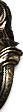## 客服服务

### 客服微信公众号微信号：changyoukf

7*24小时 全年无休

# 套路学习

#### 人族剑客

《刀剑英雄》里连招在第7，10，15，20，27，35的打击位置上是有200%的攻击加成的，所以连招被分为两类：一是以7连或10连的小连招，消耗精气少，利用加成来快速打怪。二是追求高连击数和高杀伤力的大连招，用于竞技，或打怪以造成二次掉宝。无论哪种情况，合理运用连招加成点都是很必要的。

1、技能阵法界面（F7界面）中，会显示本职业所有技能、套路、必杀。

2、点击技能阵法界面中的连续技编辑，可进入编辑连招界面。在界面中选择推荐连续技按钮，即可查看系统推荐的5种连续技。点击确定按钮，就可以自动学习该推荐连续技。

（1）小连招：
3级7连：指南决*2+乘风+指南决*4
12级7连：指南决*2+乘风+指南决*2+缠龙手（斜向）
23级10连：指南决+和合六出+缠龙手（斜向）
23级10连：指南决*2+和合六出+指南决*4
38级7连：和合六出+乘风+缠龙手（需要乘风等级6）
60级7连：指南决*2+龙腾

（2）大连招：
4级10连：如封似闭*2+指南决*2+乘风+指南决+乘风+指南决*4

8级13连：指南决*2+扫叶+指南决*2+乘风+指南决*2+如封似闭+指南决*4。

10级15连：指南决*2+扫叶+指南决*2+乘风+指南决*2+乘风+指南决*2+如封似闭+指南决+御风

16级20连：指南决*2+扫叶+指南决*2+乘风+指南决*2+乘风+指南决*2+如封似闭+指南决*2+神龙摆尾+指南决*3+御风

21级23连：指南决*2+扫叶+指南决*2+乘风+指南决*2+乘风+指南决*2+如封似闭+指南决*2+神龙摆尾+指南决*2+破玉掌+鹰扬+指南决*3+御风

23级27连：指南决*2+扫叶+指南决*2+乘风+指南决*2+乘风+指南决+和合六出+指南决*2+如封似闭+指南决*2+神龙摆尾+指南决*2+破玉掌+鹰扬+指南决*2+御风

27级32连：指南决*2+扫叶+指南决*2+乘风+指南决*2+乘风+指南决+和合六出+指南决*2+如封似闭+指南决*2+神龙摆尾+指南决*2+破玉掌+鹰扬+指南决*3+龙搏+指南决*3+御风

34级35连：指南决*2+扫叶+指南决*2+乘风+指南决*2+流星赶月+指南决+和合六出+指南决*2+如封似闭+指南决*2+神龙摆尾+指南决*2+破玉掌+鹰扬+指南决*3+龙搏+指南决*2+乘风+指南决*3+御风

37级40连：指南决*2+扫叶+指南决*2+乘风+指南决*2+流星赶月+指南决+和合六出+指南决*2+如封似闭+指南决*2+神龙摆尾+指南决*2+破玉掌+鹰扬+指南决*2+破军+指南决*2+龙搏+指南决*2+如封似闭+指南决*2+乘风+指南决*3+御风

40级44连：指南决*2+扫叶+指南决*2+乘风+指南决*2+流星赶月+指南决+和合六出+指南决*2+如封似闭+指南决*2+神龙摆尾+指南决*2+破玉掌+鹰扬+指南决*2+破军+指南决*2+龙搏+指南决*2+如封似闭+指南决*2+乘风+指南决*3+羚羊挂角+指南决*3+御风

44级49连：指南决*2+扫叶+指南决*2+乘风+指南决*2+流星赶月+指南决+和合六出+指南决*2+如封似闭+指南决*2+神龙摆尾+指南决*2+破玉掌+鹰扬+指南决*2+破军+指南决*2+龙搏+指南决*2+如封似闭+指南决*2+乘风+指南决*3+羚羊挂角+指南决*2+风卷残云+指南决*2+御风

52级54连：指南决*2+扫叶+指南决*2+乘风+指南决*2+流星赶月+指南决+和合六出+指南决*2+如封似闭+指南决*2+神龙摆尾+指南决*2+破玉掌+鹰扬+指南决*2+破军+指南决*2+龙搏+指南决*2+如封似闭+指南决*2+乘风+指南决*3+鸿飞碧落+羚羊挂角*3+指南决*2+风卷残云+指南决*2+御风

60级60连：指南决*2+扫叶+指南决*2+乘风+指南决*2+流星赶月+指南决+和合六出+指南决*2+如封似闭+指南决*2+神龙摆尾+指南决*2+破玉掌+鹰扬+指南决*2+破军+指南决*2+龙搏+指南决*2+如封似闭+指南决*2+乘风+指南决*3+鸿飞碧落+羚羊挂角*3+指南决*2+风卷残云+指南决*2+龙腾+如封似闭+乘风+御风

60级加必杀65连：指南决*2+扫叶+指南决*2+乘风+指南决*2+流星赶月+指南决+和合六出+指南决*2+如封似闭+指南决*2+神龙摆尾+指南决*2+破玉掌+鹰扬+指南决*2+破军+指南决*2+龙搏+指南决*2+如封似闭+指南决*2+乘风+指南决*3+鸿飞碧落+羚羊挂角*3+指南决*2+风卷残云+指南决*2+龙腾+（手动）骤雨打青荷

（1）小连招：

（2）大连招：
4级10连：如封似闭*2+指南决*2+乘风+指南决+乘风+指南决*4

8级13连：指南决*2+扫叶+指南决*2+乘风+指南决*2+如封似闭+指南决*4。

10级15连：指南决*2+扫叶+指南决*2+乘风+指南决*2+乘风+指南决*2+如封似闭+指南决*2+回风舞柳

16级20连：指南决*2+扫叶+指南决*2+乘风+指南决*2+乘风+指南决*2+如封似闭+指南决*2+追风+指南决*4

21级23连：指南决*2+扫叶+指南决*2+乘风+指南决*2+乘风+指南决*2+如封似闭+指南决*2+追风+指南决*2+破玉掌+鹰扬+指南决*4

23级27连：指南决*2+扫叶+指南决*2+乘风+指南决*2+乘风+指南决+和合六出+指南决*2+如封似闭+指南决*2+追风+指南决*2+破玉掌+鹰扬+指南决*2+回风舞柳

27级32连：指南决*2+扫叶+指南决*2+乘风+指南决*2+乘风+指南决+和合六出+指南决*2+如封似闭+指南决+追风+指南决*2+破玉掌+鹰扬+指南决*3+龙搏+指南决*4

34级35连：指南决*2+扫叶+指南决*2+乘风+指南决*2+流星赶月+指南决+和合六出+指南决*2+如封似闭+指南决+追风+指南决*2+破玉掌+鹰扬+指南决*3+龙搏+指南决*3+乘风+指南决*4

37级39连：指南决*2+扫叶+指南决*2+乘风+指南决*2+流星赶月+指南决+和合六出+指南决*2+如封似闭+指南决+追风+指南决*2+破玉掌+鹰扬+指南决*2+破军+指南决*2+龙搏+指南决*2+如封似闭+指南决*2+乘风+指南决*4

40级42连：指南决*2+扫叶+指南决*2+乘风+指南决*2+流星赶月+指南决+和合六出+指南决*2+如封似闭+指南决+追风+指南决*2+破玉掌+鹰扬+指南决*2+破军+指南决*2+龙搏+指南决*2+如封似闭+指南决*2+乘风+指南决*3+羚羊挂角+指南决*2+回风舞柳

44级49连：指南决*2+扫叶+指南决*2+乘风+指南决*2+流星赶月+指南决+和合六出+指南决*2+如封似闭+指南决+追风+指南决*2+破玉掌+鹰扬+指南决*2+破军+指南决*2+龙搏+指南决*2+如封似闭+指南决*2+乘风+指南决*3+羚羊挂角+指南决*2+风卷残云+指南决*4

52级54连：指南决*2+扫叶+指南决*2+乘风+指南决*2+流星赶月+指南决+和合六出+指南决*2+如封似闭+指南决+追风+指南决*2+破玉掌+鹰扬+指南决*2+破军+指南决*2+龙搏+指南决*2+如封似闭+指南决*2+乘风+指南决*3+鸿飞碧落+羚羊挂角*3+指南决*2+风卷残云+指南决*4

60级60连：指南决*2+扫叶+指南决*2+乘风+指南决*2+流星赶月+指南决+和合六出+指南决*2+如封似闭+指南决+追风+指南决*2+破玉掌+鹰扬+指南决*2+破军+指南决*2+龙搏+指南决*2+如封似闭+指南决*2+乘风+指南决*3+鸿飞碧落+羚羊挂角*3+指南决*2+风卷残云+指南决*2+龙腾+如封似闭+乘风+指南决+回风舞柳

60级加必杀65连：指南决*2+扫叶+指南决*2+乘风+指南决*2+流星赶月+指南决+和合六出+指南决*2+如封似闭+指南决+追风+指南决*2+破玉掌+鹰扬+指南决*2+破军+指南决*2+龙搏+指南决*2+如封似闭+指南决*2+乘风+指南决*3+鸿飞碧落+羚羊挂角*3+指南决*2+风卷残云+指南决*2+龙腾+（手动）骤雨打青荷